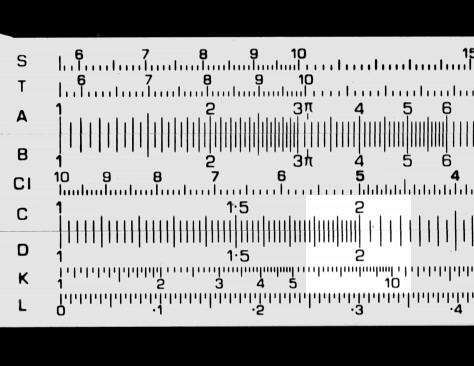Cubes and cube roots

This applies to rules with a K scale. To find the cube of a number:

• Move the cursor to the number on the D  scale.
• Read the cube of the number on the K scale.

To find the cube root, work from the K scale to the D scale.

The example below shows 2 on the D scale and its cube, 8, on the K scale.